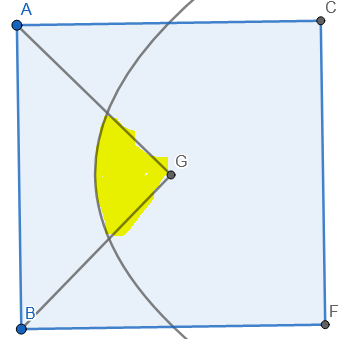# Doubt from probability

2 Likes

Anyone @Abhishek_2020_5

@Hari_Shankar Sir.
Plz see this question.

@Achyut_2020
Can you tell the source.

Ans is d?

1 Like

I don't know , a friend of mine asked me . @akash_2020 can you send your approach please , i don't know ans eitherthe probability is the area of yellow region when the side length of square is one
so convert the system into coordinates with B as origin
so the equation of BG is y = x and AG is y = 1-x
and equation of parabola is (y-\frac{1}{2})^2 =x - \frac{1}{2}
which now can be easily solved

8 Likes

Hint: It will be the area common to the interior of parabolas formed with centre as focus and the sides of the square as directrix

1 Like